# Exponential Functions & Logarithmic Functions: Homework Help Chapter Exam

Exam Instructions:

Choose your answers to the questions and click 'Next' to see the next set of questions. You can skip questions if you would like and come back to them later with the yellow "Go To First Skipped Question" button. When you have completed the practice exam, a green submit button will appear. Click it to see your results. Good luck!

### Page 1

#### Question 1 1. Evaluate.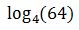#### Question 5 5. Condense the logarithm below: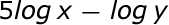### Page 2

#### Question 6 6. Solve the differential equation for your bank balance given as follows.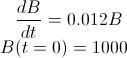#### Question 7 7. Evaluate the logarithm below: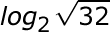#### Question 8 8. What transformation happens to this function?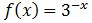#### Question 9 9. The following differential equation represents the change in population as a function of time. What does the 0.03 represent in this equation?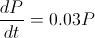### Page 3

#### Question 12 12. Simplify the following expression.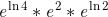#### Question 14 14. What kind of shift happens with this function?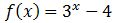#### Question 15 15. What does this equal?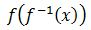### Page 4

#### Question 16 16. Solve the following equation for y = f(x).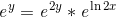#### Question 19 19. What transformation happens to this function?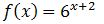### Page 5

#### Question 22 22. What transformation happens with this function?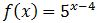#### Question 25 25. Solve the following equation for x.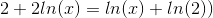### Page 6

#### Question 30 30. Simplify: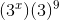#### Exponential Functions & Logarithmic Functions: Homework Help Chapter Exam Instructions

Choose your answers to the questions and click 'Next' to see the next set of questions. You can skip questions if you would like and come back to them later with the yellow "Go To First Skipped Question" button. When you have completed the practice exam, a green submit button will appear. Click it to see your results. Good luck!

Support